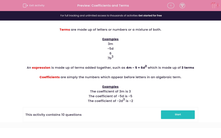# Coefficients and Terms

In this worksheet, students identify coefficients and terms in algebraic expressions.Key stage:  KS 2

Curriculum topic:   Maths and Numerical Reasoning

Curriculum subtopic:   Equations and Algebra

Difficulty level:#### Worksheet Overview

Terms are made up of letters or numbers or a mixture of both.

Examples

3m

-5d

6

7b3

An expression is made up of terms added together, such as 4m - 5 + 6d2 which is made up of 3 terms

Coefficients are simply the numbers which appear before letters in an algebraic term.

Examples

The coefficient of 3m is 3

The coefficient of -5d is -5

The coefficient of -2d3 is -2

### What is EdPlace?

We're your National Curriculum aligned online education content provider helping each child succeed in English, maths and science from year 1 to GCSE. With an EdPlace account you’ll be able to track and measure progress, helping each child achieve their best. We build confidence and attainment by personalising each child’s learning at a level that suits them.

Get started